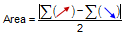## 3. Area Computation

The area of a non-crossing closed polygon, Figure C-5,Figure C-5 Polygon Areas

can be computed using the coordinates of its vertices:Equation C-5

In surveying terms using North and East coordinates:Equation C-6

An easy way to remember either equation is graphically:

 X & Y E & N List coordinates in order around exterior. Repeat first coordinate pair at end.Cross multiply.Sum cross-productsAdd the cross multiplication sums, divide by two, and take the absolute value to obtain the area:Equation C-7

The absolute value is used because area could be positive or negative depending on traverse configuration, direction around it, and coordinate order (eg, E & N or N & E). Positive or negative, the area magnitude will be correct.